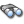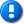alvinalexander.com | career | drupal | java | mac | mysql | perl | scala | uml | unix

# Java example source code file (StepFunction.java)

This example Java source code file (StepFunction.java) is included in the alvinalexander.com "Java Source Code Warehouse" project. The intent of this project is to help you "Learn Java by Example" TM.

## Java - Java tags/keywords

dimensionmismatchexception, nodataexception, nonmonotonicsequenceexception, nullargumentexception, stepfunction, univariatefunction, util

## The StepFunction.java Java example source code

```/*
* Licensed to the Apache Software Foundation (ASF) under one or more
* contributor license agreements.  See the NOTICE file distributed with
* The ASF licenses this file to You under the Apache License, Version 2.0
* (the "License"); you may not use this file except in compliance with
* the License.  You may obtain a copy of the License at
*
*
* Unless required by applicable law or agreed to in writing, software
* WITHOUT WARRANTIES OR CONDITIONS OF ANY KIND, either express or implied.
* See the License for the specific language governing permissions and
*/

package org.apache.commons.math3.analysis.function;

import java.util.Arrays;

import org.apache.commons.math3.analysis.UnivariateFunction;
import org.apache.commons.math3.exception.DimensionMismatchException;
import org.apache.commons.math3.exception.NoDataException;
import org.apache.commons.math3.exception.NonMonotonicSequenceException;
import org.apache.commons.math3.exception.NullArgumentException;
import org.apache.commons.math3.util.MathArrays;

/**
* <a href="http://en.wikipedia.org/wiki/Step_function">
*  Step function</a>.
*
* @since 3.0
*/
public class StepFunction implements UnivariateFunction {
/** Abscissae. */
private final double[] abscissa;
/** Ordinates. */
private final double[] ordinate;

/**
* Builds a step function from a list of arguments and the corresponding
* values. Specifically, returns the function h(x) defined by <pre>```
* h(x) = y for all x < x
*        y for x ≤ x < x
*        ...
*        y[y.length - 1] for x ≥ x[x.length - 1]
* </code>``````
``` * The value of {@code x} is ignored, but it must be strictly less than * {@code x}. * * @param x Domain values where the function changes value. * @param y Values of the function. * @throws NonMonotonicSequenceException * if the {@code x} array is not sorted in strictly increasing order. * @throws NullArgumentException if {@code x} or {@code y} are {@code null}. * @throws NoDataException if {@code x} or {@code y} are zero-length. * @throws DimensionMismatchException if {@code x} and {@code y} do not * have the same length. */ public StepFunction(double[] x, double[] y) throws NullArgumentException, NoDataException, DimensionMismatchException, NonMonotonicSequenceException { if (x == null || y == null) { throw new NullArgumentException(); } if (x.length == 0 || y.length == 0) { throw new NoDataException(); } if (y.length != x.length) { throw new DimensionMismatchException(y.length, x.length); } MathArrays.checkOrder(x); abscissa = MathArrays.copyOf(x); ordinate = MathArrays.copyOf(y); } /** {@inheritDoc} */ public double value(double x) { int index = Arrays.binarySearch(abscissa, x); double fx = 0; if (index < -1) { // "x" is between "abscissa[-index-2]" and "abscissa[-index-1]". fx = ordinate[-index-2]; } else if (index >= 0) { // "x" is exactly "abscissa[index]". fx = ordinate[index]; } else { // Otherwise, "x" is smaller than the first value in "abscissa" // (hence the returned value should be "ordinate"). fx = ordinate; } return fx; } } Other Java examples (source code examples) Here is a short list of links related to this Java StepFunction.java source code file:The search pageOther Java source code examples at this package levelClick here to learn more about this project ```
 ... this post is sponsored by my books ...#1 New Release!FP Best Seller